# Polynomial Representation using Linked List in C

## Polynomial Representation using Linked List in C Language:

In this article, I am going to discuss Polynomial Representation using Linked List in C Language with Examples. Please read our previous article, where we discussed Sparse Matrix using Linked List in C Language with Examples.

##### Polynomial Representation using Linked List in C:

In this article, we will learn about polynomial representation by using a linked list. We have already covered this topic in arrays. If you have not seen that article then you can read that here. Below is an example of a polynomial.

P(x) = 4x3 + 9x2 + 6x + 7

This is a univariate polynomial. It is defined in terms of just a single variable ‘x’. It is the summation of terms. There is more than one term being added together. If we know the value of ‘x’ we can evaluate and get a result of this polynomial.

We want to represent this in our applications. So, for representation, we have to store the data about that polynomial. That data can be stored either in an array or a linked list. So, we have already seen array representation. Now we will see how to represent the data related to polynomials. If we observe the above polynomial, each term is having its coefficient and exponent.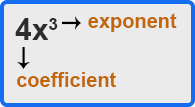If we know the value of x then we can raise the power of x or the exponent of x and then multiply with the coefficient. So, in this way, we can get the answer for one term. Likewise, we can evaluate all the terms and get the result. It is sufficient to store the coefficient and exponent of each term. We can represent these terms in the form of a linked list. So, we can define each term as a node and we will have a set of nodes for a single polynomial. So let us define a node for a single term.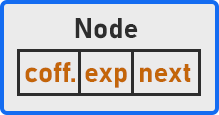Here is the node that is having a coefficient, an exponent, and a pointer (next) to the next node. Let us define the structure for this.

```struct Node {
int coefficient;
int exponent;
struct Node *next;
}
```

This structure has a coefficient and exponent of type int and a pointer to the next node of type Node*. If the coefficient is in decimal, then you can take float also. Now one more thing you can observe, this is the node of the linked list and it is having 3 members. So, the linked list that we have studied was taking only one value but now we are using a linked list. Based on the requirements a node can have any number of data members. Now, let us represent the polynomial as a linked list.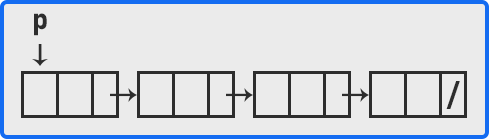Here we have a linked type Node which we have created above. Each node has 3 sections: coefficient, exponent, and a pointer to the next node. There are 4 terms in the above polynomial so we have taken 4 nodes here. Let us fill up the value from the above polynomial.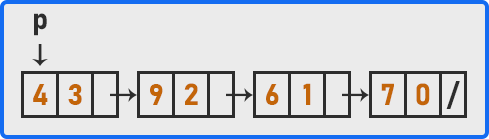This is how a polynomial is represented in the form of a linked list. In array representation, we were storing the number of terms but here we do not need to store. If you want then you can store that otherwise, the number of nodes is equal to the total number of terms. Here, we will not have extra nodes. Now we will learn how to evaluate the polynomial. We have stored all the required data to evaluate a polynomial value but one more thing is required which is the value of ‘x’.

P(x) = 4x3 + 9x2 + 6x + 7

If suppose the value of ‘x’ is 1 then,

P (x) = (4 * 13) + (9 * 12) + (6 * 1) + 7

P (x) = 4 + 9 + 6 + 7

P (x) = 26

If the value of ‘x’ is 2 then,

P(x) = (4 * 23) + (9 * 22) + (6 * 2) + 7

So, with the value of x, we can evaluate the result of the polynomial. Let us look at the procedure of evaluation. Suppose the value of ‘x’ is 2 then, we have to raise the power of x and then multiply it with the coefficient. This will give the result of a single term. Then we have to add the result of the previous term with the result of the next term. So, we have to add the result of all the terms to get the result. We will take a variable i.e. sum which will store the addition of the result of all the previous terms. For example,

P (x) = 4x3 + 9x2 + 6x + 7

Sum = 0;

To evaluate 4x3,

4 * 23 = 32

Sum = 0 + 32 = 32

To evaluate 9x2,

9 * 22 = 36

Sum = 32 + 36 = 68

To evaluate 6x,

6 * 2 = 12

Sum = 68 + 12 = 80

To evaluate 7,

Sum = 80 + 7 = 87

So, when we put the value of ‘x’ as 2 then we will get 87 as the result of the polynomial. Now we will write a function for evaluation.

```double evaluate (int x){
double sum = 0.0;
Node *q = p;

while(q != NULL){
sum += q->coff * pow(x, q->exp);
q = q->next;
}
return sum;
}
```

So, this evaluate function will take the value of ‘x’ as a parameter and it will traverse the nodes of the linked list, and at each node, it will store the sum of all the previous nodes or terms and at last, it will return the sum. Now let us look at the complete program.

##### C Program to understand the Evaluation of polynomials using Linked List Representation:
```#include <stdio.h>
#include <stdlib.h>
#include <math.h>

struct Node {
int coeff;
int exp;
struct Node * next;
}* poly = NULL;

void create() {
struct Node * t, * last = NULL;
int num, i;

printf("Enter number of terms: ");
scanf("%d", & num);
printf("Enter each term with coeff and exp:\n");

for (i = 0; i < num; i++) {
t = (struct Node * ) malloc(sizeof(struct Node));
scanf("%d%d", & t -> coeff, & t -> exp);
t -> next = NULL;
if (poly == NULL) {
poly = last = t;
} else {
last -> next = t;
last = t;
}
}
}

void Display(struct Node * p) {
printf("%dx%d ", p -> coeff, p -> exp);
p = p -> next;

while (p) {
printf("+ %dx%d ", p -> coeff, p -> exp);
p = p -> next;
}
printf("\n");
}

long Eval(struct Node * p, int x) {
long val = 0;

while (p) {
val += p -> coeff * pow(x, p -> exp);
p = p -> next;
}

return val;
}

int main() {
int x;
create();
Display(poly);

printf("Enter value of x: ");
scanf("%d", &x);

printf("%ld\n", Eval(poly, x));
return 0;
}
```
###### Output: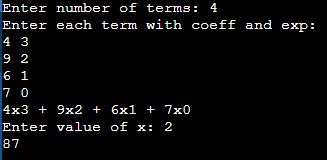In the next article, I am going to discuss Polynomial Representation using Linked List in C Language. Here, in this article, I try to explain Polynomial Representation using Linked List in C Language with Examples. I hope you enjoy this Polynomial Representation using the Linked List article.